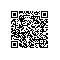# 10分钟让你彻底了解 Java 8 的 Lambda、函数式接口、Stream 用法和原理

10分钟让你彻底了解 Java 8 的 Lambda、函数式接口、Stream 用法和原理

Java 8 是在 2014 年发布的，实话说，风筝我也是在 Java 8 发布后很长一段时间才用的 Stream，因为 Java 8 发布的时候我还在 C# 的世界中挣扎，而使用 Lambda 表达式却很早了，因为 Python 中用 Lambda 很方便，没错，我写 Python 的时间要比 Java 的时间还长。

Lambda 表达式
Lambda 表达式是一个匿名函数，Lambda表达式基于数学中的λ演算得名，直接对应于其中的lambda抽象，是一个匿名函数，即没有函数名的函数。Lambda表达式可以表示闭包。

// 无参数，无返回值
() -> log.info("Lambda")

// 有参数，有返回值
(int a, int b) -> { a+b }

log.info("Lambda");

private int plus(int a, int b){

  return a+b;

}

@Override
public void run() {
System.out.println("快速新建并启动一个线程");
}

}).run();

System.out.println("快速新建并启动一个线程");

}).run();

Lambda 表达式简化了匿名内部类的形式，可以达到同样的效果，但是 Lambda 要优雅的多。虽然最终达到的目的是一样的，但其实内部的实现原理却不相同。

Function s = Integer::parseInt;
Integer i = s.apply("10");

Comparator comparator = Integer::compare;
int result = comparator.compare(100,10);

IntBinaryOperator intBinaryOperator = Integer::compare;
int result = intBinaryOperator.applyAsInt(10,100);Q：什么样的方法可以被引用？

A：这么说吧，任何你有办法访问到的方法都可以被引用。

Q：返回值到底是什么类型？

A：这就问到点儿上了，上面又是 Function、又是Comparator、又是 IntBinaryOperator的，看上去好像没有规律，其实不然。

@FunctionalInterface
public interface Function {

R apply(T t);

}

public static int parseInt(String s) throws NumberFormatException {

return parseInt(s,10);

}

Integer.compare方法定义如下：

public static int compare(int x, int y) {

return (x < y) ? -1 : ((x == y) ? 0 : 1);

}

@FunctionalInterface
public interface Comparator {

int compare(T o1, T o2);

}

@FunctionalInterface
public interface IntBinaryOperator {

int applyAsInt(int left, int right);

}

JDK 中定义了很多函数式接口，主要在 java.util.function包下，还有 java.util.Comparator 专门用作定制比较器。另外，前面说的 Runnable也是一个函数式接口。1. 定义一个函数式接口，并添加一个方法

@FunctionalInterface
public interface KiteFunction {

/**
* 定义一个双参数的方法
* @param t
* @param s
* @return
*/
R run(T t,S s);

}

1. 定义一个与 KiteFunction 中 run 方法对应的方法

public class FunctionTest {

public static String DateFormat(LocalDateTime dateTime, String partten) {
DateTimeFormatter dateTimeFormatter = DateTimeFormatter.ofPattern(partten);
return dateTime.format(dateTimeFormatter);
}

}
3.用方法引用的方式调用

KiteFunction functionDateFormat = FunctionTest::DateFormat;
String dateString = functionDateFormat.run(LocalDateTime.now(),"yyyy-MM-dd HH:mm:ss");

public static void main(String[] args) throws Exception {

String dateString = new KiteFunction<LocalDateTime, String, String>() {
@Override
public String run(LocalDateTime localDateTime, String s) {
DateTimeFormatter dateTimeFormatter = DateTimeFormatter.ofPattern(s);
return localDateTime.format(dateTimeFormatter);
}
}.run(LocalDateTime.now(), "yyyy-MM-dd HH:mm:ss");
System.out.println(dateString);

}

public static void main(String[] args) throws Exception {

    KiteFunction<LocalDateTime, String, String> functionDateFormat = (LocalDateTime dateTime, String partten) -> {
DateTimeFormatter dateTimeFormatter = DateTimeFormatter.ofPattern(partten);
return dateTime.format(dateTimeFormatter);
};
String dateString = functionDateFormat.run(LocalDateTime.now(), "yyyy-MM-dd HH:mm:ss");
System.out.println(dateString);

}

Stream API

Collection接口提供了 stream()方法，让我们可以在一个集合方便的使用 Stream API 来进行各种操作。值得注意的是，我们执行的任何操作都不会对源集合造成影响，你可以同时在一个集合上提取出多个 stream 进行操作。

public interface Stream extends BaseStream> {

Stream filter(Predicate<? super T> predicate);

// 其他接口
}

of

private static void createStream(){

Stream<String> stringStream = Stream.of("a","b","c");

}
empty

concat

private static void concatStream(){

Stream<String> a = Stream.of("a","b","c");
Stream<String> b = Stream.of("d","e");
Stream<String> c = Stream.concat(a,b);

}
max

private static void max(){

Stream<Integer> integerStream = Stream.of(2, 2, 100, 5);
Integer max = integerStream.max(Integer::compareTo).get();
System.out.println(max);

}

private static void max(){

Stream<Integer> integerStream = Stream.of(2, 2, 100, 5);
Comparator<Integer> comparator =  (x, y) -> (x.intValue() < y.intValue()) ? -1 : ((x.equals(y)) ? 0 : 1);
Integer max = integerStream.max(comparator).get();
System.out.println(max);

}
min

findFirst

findAny

count

Stream a = Stream.of("a", "b", "c");
long x = a.count();
peek

private static void peek() {

Stream<String> a = Stream.of("a", "b", "c");
List<String> list = a.peek(e->System.out.println(e.toUpperCase())).collect(Collectors.toList());

}
forEach

private static void forEach() {

Stream<String> a = Stream.of("a", "b", "c");
a.forEach(e->System.out.println(e.toUpperCase()));

}
forEachOrdered

Stream a = Stream.of("a", "b", "c");
a.parallel().forEach(e->System.out.println(e.toUpperCase()));

Stream a = Stream.of("a", "b", "c");
a.parallel().forEachOrdered(e->System.out.println(e.toUpperCase()));
limit

private static void limit() {

Stream<String> a = Stream.of("a", "b", "c");
a.limit(2).forEach(e->System.out.println(e));

}

skip

private static void skip() {

Stream<String> a = Stream.of("a", "b", "c");
a.skip(2).forEach(e->System.out.println(e));

}
distinct

private static void distinct() {

Stream<String> a = Stream.of("a", "b", "c","b");
a.distinct().forEach(e->System.out.println(e));

}
sorted

private static void sorted() {

Stream<String> a = Stream.of("a", "c", "b");
a.sorted().forEach(e->System.out.println(e));

}

private static void sortedWithComparator() {

Stream<String> a = Stream.of("a1", "c6", "b3");
a.sorted((x,y)->Integer.parseInt(x.substring(1))>Integer.parseInt(y.substring(1))?1:-1).forEach(e->System.out.println(e));

}

private static List getUserData() {

Random random = new Random();
List<User> users = new ArrayList<>();
for (int i = 1; i <= 10; i++) {
User user = new User();
user.setUserId(i);
user.setAge(random.nextInt(100));
user.setGender(i % 2);
user.setPhone("18812021111");
}
return users;

}
filter

private static void filter(){

List<User> users = getUserData();
Stream<User> stream = users.stream();
stream.filter(user -> user.getGender().equals(0) && user.getAge()>50).forEach(e->System.out.println(e));

/**
*等同于下面这种形式 匿名内部类
*/

// stream.filter(new Predicate() {
// @Override
// public boolean test(User user) {
// return user.getGender().equals(0) && user.getAge()>50;
// }
// }).forEach(e->System.out.println(e));
}
map
map方法的接口方法声明如下，接受一个 Function函数式接口，把它翻译成映射最合适了，通过原始数据元素，映射出新的类型。

Stream map(Function<? super T, ? extends R> mapper);

@FunctionalInterface
public interface Function {

/**
* Applies this function to the given argument.
*
* @param t the function argument
* @return the function result
*/
R apply(T t);

}

private static void map(){

List<User> users = getUserData();
Stream<User> stream = users.stream();
List<UserDto> userDtos = stream.map(user -> dao2Dto(user)).collect(Collectors.toList());

}

private static UserDto dao2Dto(User user){

UserDto dto = new UserDto();
BeanUtils.copyProperties(user, dto);
//其他额外处理
return dto;

}
mapToInt

mapToLong

mapToDouble

flatMap

Stream
Stream>
Stream>

private static void flatMap(){

List<User> users = getUserData();
List<User> users1 = getUserData();
List<List<User>> userList = new ArrayList<>();
Stream<List<User>> stream = userList.stream();
List<UserDto> userDtos = stream.flatMap(subUserList->subUserList.stream()).map(user -> dao2Dto(user)).collect(Collectors.toList());

}
flatMapToInt

flatMapToLong

flatMapToDouble

collection

R collect(Collector<? super T, A, R> collector);

private static void collect(){

Stream<Integer> integerStream = Stream.of(1,2,5,7,8,12,33);
List<Integer> list = integerStream.filter(s -> s.intValue()>7).collect(Collectors.toList());

}

private static void collect(){

Stream<Integer> integerStream = Stream.of(1,2,5,7,8,12,33);
List<Integer> list = integerStream.filter(s -> s.intValue()>7).collect(ArrayList::new, ArrayList::add,
ArrayList::addAll);

}

// 返回 userId:List
Map> map = user.stream().collect(Collectors.groupingBy(User::getUserId));

// 返回 userId:每组个数
Map map = user.stream().collect(Collectors.groupingBy(User::getUserId,Collectors.counting()));
toArray
collection是返回列表、map 等，toArray是返回数组，有两个重载，一个空参数，返回的是 Object[]。

@FunctionalInterface
public interface IntFunction {

/**
* Applies this function to the given argument.
*
* @param value the function argument
* @return the function result
*/
R apply(int value);

}

private static void toArray() {

List<User> users = getUserData();
Stream<User> stream = users.stream();
User[] userArray = stream.filter(user -> user.getGender().equals(0) && user.getAge() > 50).toArray(User[]::new);

}
reduce

private static void reduce(){

Stream<Integer> integerStream = Stream.of(1,2,5,7,8,12,33);
Integer sum = integerStream.reduce(0,(x,y)->x+y);
System.out.println(sum);

}

Stream 本质上来说就是用来做数据处理的，为了加快处理速度，Stream API 提供了并行处理 Stream 的方式。通过 users.parallelStream()或者users.stream().parallel() 的方式来创建并行 Stream 对象，支持的 API 和普通 Stream 几乎是一致的。

CPU 密集型计算适合使用并行 Stream，而 IO 密集型使用并行 Stream 反而会更慢。

Java 25 周岁了，有多少同学跟我一样在用 Java 8，还有多少同学再用更早的版本，请说出你的故事。使用钉钉扫一扫加入圈子
+ 订阅